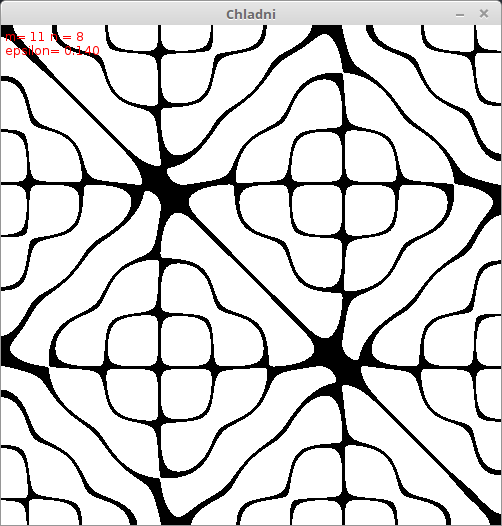### Paul Bourke has created fantastic stuff

Here is a processing sketch by Julien Gachadoat aka v3ga based on Paul Bourkes work translated for JRubyArt by Martin Prout

``````# Chladni plate interference surfaces
# —
# Based on :
#
# —
# Developed on:
#   - Processing 2.1.1 on MacOSX (10.9.2)
# www.v3ga.net
# www.2roqs.com
#
# Translated and tested on:
#   - JRubyArt-1.4.6
# Martin Prout

def settings
size(500, 500, P2D)
end

def setup
@epsilon = 0.05
@recompute = true
@m = 10
@n = 2
end

def draw
return unless recompute
@recompute = false
background(255)
grid(width, height) do |x, y|
chladni = cos(n * PI * x / width) * cos(m * PI * y / width) - cos(m * PI * x / width) * cos(n * PI * y / width)
pixels[x + y * width] = 0 if chladni.abs <= epsilon
end
update_pixels
format_string = "m= %d n = %d \nepsilon= %0.3f"
infos = format(format_string, m, n, epsilon)
fill(255, 0, 0)
text(infos, 4, 16)
end

def key_pressed
case key
when '+'
@epsilon += 0.01
when '-'
@epsilon -= 0.01
when CODED
case key_code
when UP
@m += 1
when DOWN
@m -= 1
when LEFT
@n -= 1
when RIGHT
@n += 1
end
end
@recompute = true
@m = constrain(m, 1, 20)
@n = constrain(n, 1, 20)
end

``````